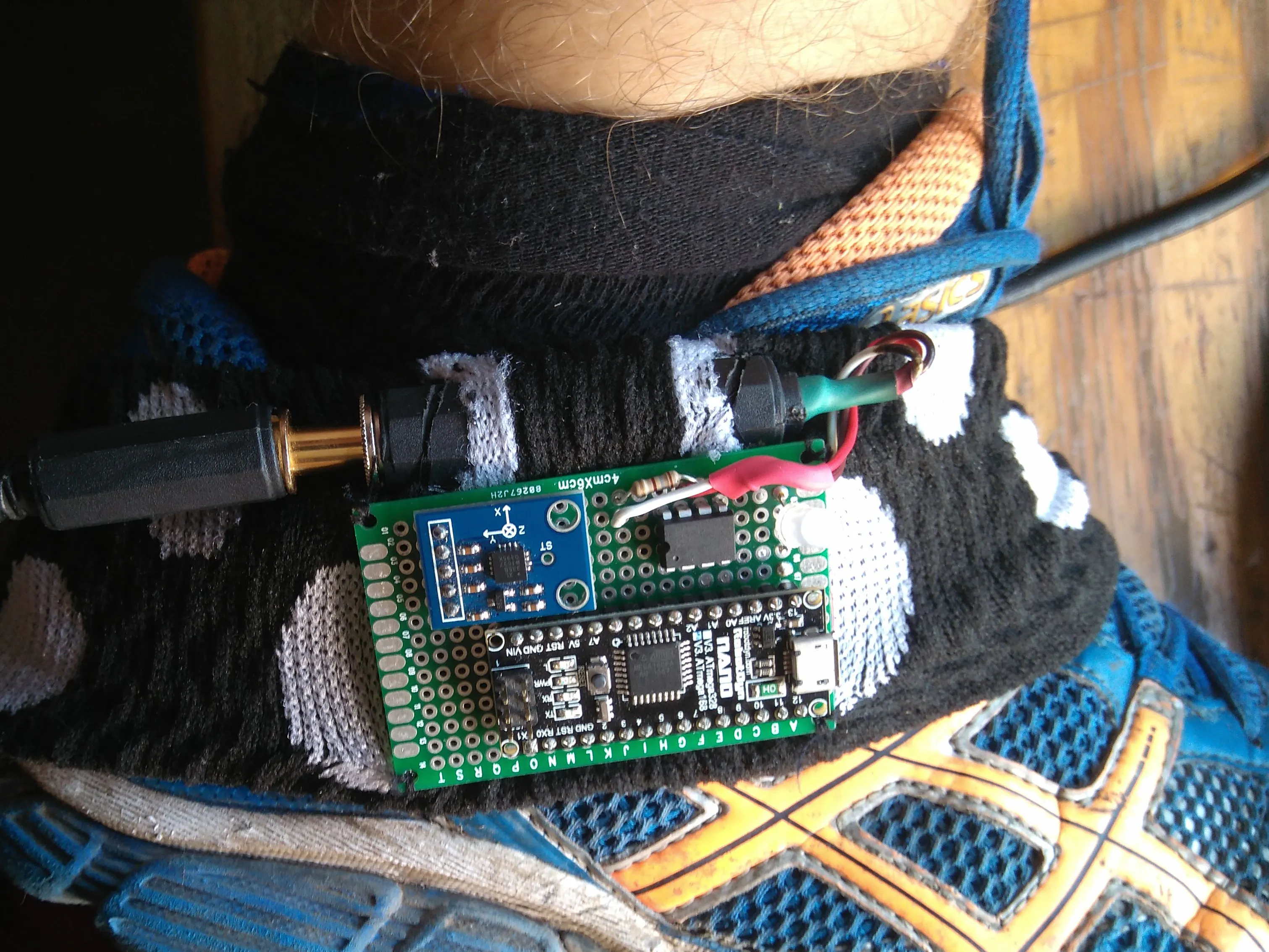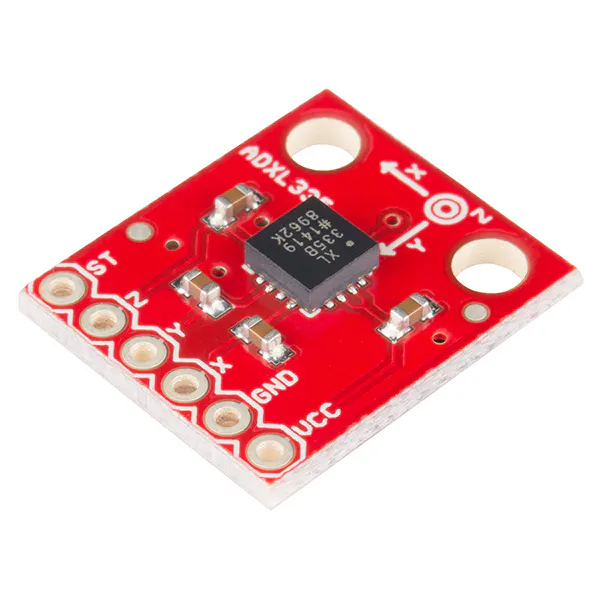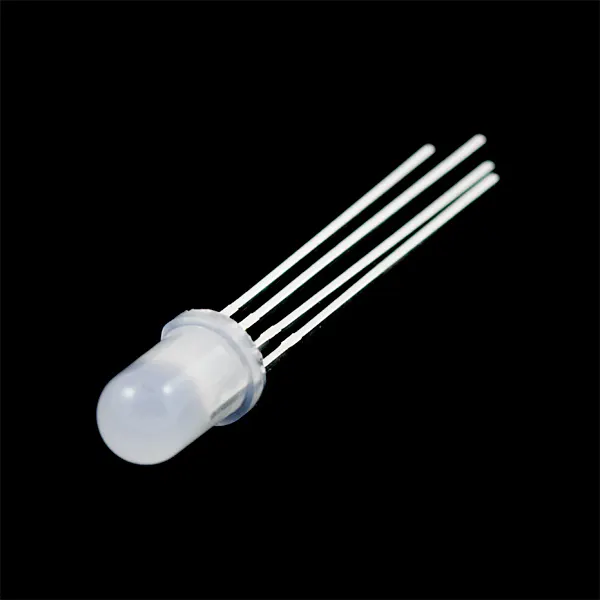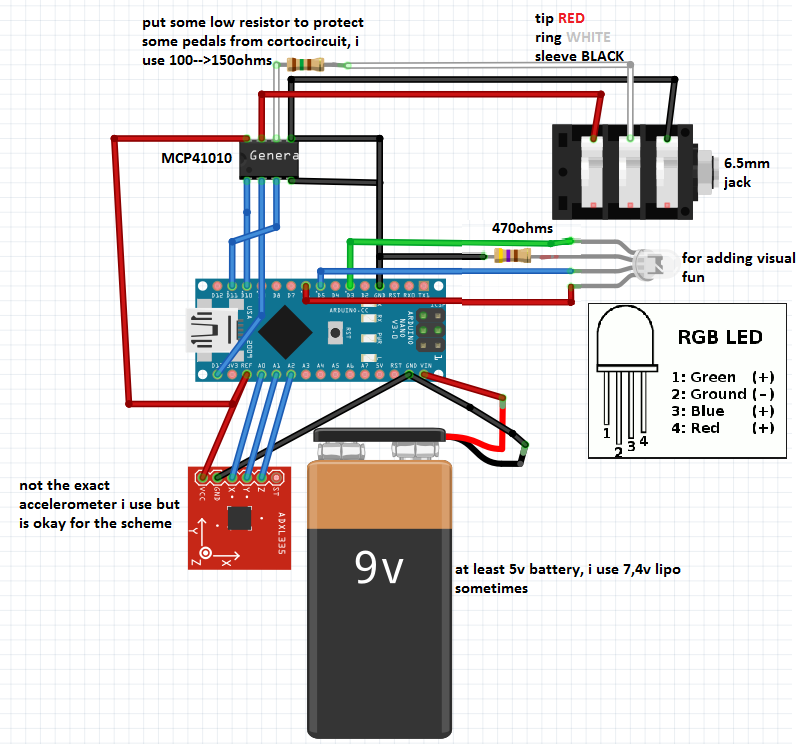Project in progress# Ankle Air Guitar Pedal © MIT

Build an "invisibe" expression pedal for your guitar with MCP41010 and Arduino Nano. It's awesome and simple. May the funk be with you.

• 8,069 views
• 25 respects

## Components and suppliesArduino Nano R3 any arduino is okay, im thinking on using tinyduino
×1
 mpc41010 digital potenciometer
×1SparkFun Triple Axis Accelerometer Breakout - ADXL335 any analogoutput accelerometer is okay
×1
 stereo audio jack 6,5 mm female adapter
×1
 stereo audio jack 6,5 mm cable
×1RGB Diffused Common Cathode just for fun, no needed
×1

## Apps and online services

We need to weld the correct things, so follow along. The current interest in dubstep was growing, and I was ready to spend 40€ on one expression pedal for my boss synthesizer when I remembered I was an electronics nerd.

Then science occurred.

At first, I thought about drilling a shoe and gluing the electronics inside, but I did not have a pair of shoes to spare. So, I ended up purchasing a hair band and stitching thread.

### First steps with the MPC digital potentiometers family:

We have a lot of types to choose from, but I picked this one - the MPC41010.

• MPC41010: single potentiometer, 10k ohm
• MPC41050: single potentiometer, 50k ohm
• MPC41100: single potentiometer, 100k ohm
• MCP42010: two independent potentiometers, 10k ohm
• MCP42050: two independent potentiometers, 50k ohm
• MCP42100: two independent potentiometers, 100k ohm

More things about them here. Important things that you need to know for hacking this device:

• You can do similar things with other digital potentiometers. (like the COM-10613, more info)
• The resistance is 0 to 10k ohms divided in 255 steps with each step of 39 ohms. (MATH!)
• Serial communication: just use `#include <SPI.h>`.

### First Prototype: Lessons Learned

I love the smell of recycled cardboard in the morning.

What I learned with the prototype:

• When the device is reset, the human needs to set the flat and the top values for adjusting the range of movement.
• It would be nice to implement a function that chooses between the axis which had the wider range of variation. This would be useful so that you don't have to mind the position of the accelerometer; you could put it in upside-down, on the bass top, on your wrist, etc.
• Maybe I need to implement a logarithmic behaviour for the resistance values.
• I need to take care of the 0 level because it is highly instable.

### Building the stereo jack 6,5 mm cable

Keep in mind I am poor. So for 4€, I bought the components (couple of male jack endings and 1 meter of cable).

We need to weld the correct things, so follow the scheme and use some hot-shrink tube to do a fine job.

## Code

##### MAIN THINGArduino
main raw program, the rgb led or any pwm signal is catastrophic fro the accelerometer readings (noise)
```#include <SPI.h>
#define debug //comment this line if you´re not gonna use the serial data with the arduino ide

#define CS 10 //chipselect

#define accelerometerX A0//analogpins for the accelerometer
#define accelerometerY A1
#define accelerometerZ A2

#define RED 6 //rgb pins
#define GREEN 3
#define BLUE 5

//MCP41010 chip´s connection
//        39ohms per step of the
//CS  10              U      vcc
//SCK 13 serial clock        PBO--UPRESISTOR
//SI  11 serial imput        PWO  INRESISTOR
//gnd                        PAO--BOTTOMRESISTOR

int datarray={0,0,0,0,0,0,1023,1023,1023,0,0,0,0,0,0};
int smoothingarray={0,0,0,0,0,0,0,0,0,0,0,0,0,0,0};
int rgb;
int i=0,chipvalue=0;
int ResistorValue=0;
void setup() {
//
//pinMode (GREEN, OUTPUT);//GREEN RGB (PWM)
//pinMode (BLUE, OUTPUT);//BLUE
//pinMode (RED, OUTPUT);//RED
//digitalWrite(GREEN, HIGH);//setting the led off at the begining
//digitalWrite (BLUE, HIGH);//
//digitalWrite (RED,  HIGH);//

pinMode (CS, OUTPUT);//chipselect for the  MCP41010 chip
pinMode (13, OUTPUT);//things are happening led
pinMode (accelerometerX, INPUT);//acelerometer´s inputs
pinMode (accelerometerY, INPUT);//
pinMode (accelerometerZ, INPUT);//
SPI.begin();
MCP41010Write(0);//init value horizontal foot

#ifdef debug
Serial.begin(9600);
#endif
}

void loop() {

comparemaxmin(datarray);

detectRange(datarray);

ResistorValue=map(datarray[datarray],datarray[datarray+6]+20,datarray[datarray+3]-10,0,255);//+20 to the min and -10 to the max for preventing unreachable edges

chipvalue =constrain(ResistorValue,0,255);

MCP41010Write(smoothing(chipvalue));

//  //map(value, fromLow, fromHigh, toLow, toHigh)
// for(i=0;i<=2;i++){
//  rgb[i]=map(datarray[i],datarray[i+6],datarray[i+3],0,255);
// }
////analogWrite(GREEN, rgb);//EL LED DA PROBLEMAS DE LECTURA ruido/////////////////////7
////analogWrite (BLUE, rgb);//
////analogWrite (RED,  rgb);//

#ifdef debug
Serial.print(datarray);
Serial.print(" ");
Serial.print(datarray);
Serial.print(" ");
Serial.print(datarray);

Serial.print("     /max ");
Serial.print(datarray);
Serial.print(" ");
Serial.print(datarray);
Serial.print(" ");
Serial.print(datarray);

Serial.print(" /min ");
Serial.print(datarray);
Serial.print(" ");
Serial.print(datarray);
Serial.print(" ");
Serial.print(datarray);

Serial.print("    //GAP :");
Serial.print(datarray);
Serial.print(" ");
Serial.print(datarray);
Serial.print(" ");
Serial.print(datarray);

//      Serial.print("/?? ");
//      Serial.print(datarray);
//      Serial.print(" ");
//      Serial.print(datarray);
//      Serial.print(" ");
//      Serial.print(datarray);

Serial.print("  //axis choose ");
Serial.print(datarray);

Serial.print("       //chip value ");
Serial.print(chipvalue);

Serial.println();
#endif
delay(100);//delay for stability
}

void comparemaxmin(int datarray[])
{
for(i=0;i<=2;i++)
datarray[i+3]=max(datarray[i],datarray[i+3]);

for(i=0;i<=2;i++)
datarray[i+6]=min(datarray[i],datarray[i+6]);

}

void detectRange(int datarray)
{
for(i=0;i<=2;i++)
{
datarray[i+9]=abs(abs(datarray[i+3])-abs(datarray[i+6]));
}
if(datarray>=datarray&&datarray>=datarray)//choosing the axis with the wider range
datarray=0;
if(datarray>=datarray&&datarray>=datarray)
datarray=1;
if(datarray>=datarray&&datarray>=datarray)
datarray=2;
}

void MCP41010Write(byte value) //speaking protocol with the mcp chip
{
// Note that the integer value passed to this subroutine
// is cast to a byte

digitalWrite(CS,LOW);
SPI.transfer(B00010001); // This tells the chip to set the pot
SPI.transfer(value);     // This tells it the pot position
digitalWrite(CS,HIGH);
}

int smoothing(int data){
int result,j;

for(j=0;j<=14;j++){
smoothingarray[j] =  smoothingarray[j+1];
}
smoothingarray= data;

result=(smoothingarray+smoothingarray+smoothingarray+smoothingarray+smoothingarray)/5;
constrain(result,0,255);
return result;
}
```

## Schematics#### Arduino UNO Guitar Pedal

Project tutorial by electrosmash

• 94,417 views
• 244 respects

#### Arduino MEGA Guitar Pedal

Project tutorial by electrosmash

• 33,198 views
• 140 respects

#### Arduino DUE Programmable Guitar Pedal

Project showcase by Team ElectroSmash Team.

• 22,071 views
• 66 respects

#### Turn an Arduino Uno into a MIDI Controller: Guitar Pedals

Project tutorial by Johan van Vugt

• 19,769 views
• 16 respects

#### Guitar Speed Pick and Stomp Pedal!

Project tutorial by Marc Uberstein

• 8,755 views